# Algebra of Complex Numbers Notes | Study Mathematics (Maths) Class 11 - JEE

## JEE: Algebra of Complex Numbers Notes | Study Mathematics (Maths) Class 11 - JEE

The document Algebra of Complex Numbers Notes | Study Mathematics (Maths) Class 11 - JEE is a part of the JEE Course Mathematics (Maths) Class 11.
All you need of JEE at this link: JEE

Complex Numbers

A. Definition
Complex numbers are defined as expressions of the form a + ib where a , b ∈ R &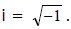It is denoted by z i.e. z = a + ib . ‘a’ is called as real part of z (Re z) and ‘b’ is called as imaginary part of z (Im z).

Every Complex Number Can Be Regarded As

Purely real  -  Purely imaginary - Imaginary
if b = 0    -   if a = 0  -  if b ≠ 0

Remark :
(a) The set R of real numbers is a proper subset of the complex numbers . Hence the complete number system is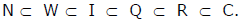(b) Zero is both purely real as well as purely imaginary but not imaginary .
(c)is called the imaginary unit . Also i² = - l ; i3 = -i ; i4 = 1 etc.
(d)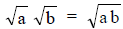only if atleast one of either a or b is non - negative.

B. Algebraic Operations
The algebraic operations on complex numbers are similar to those on real numbers treating ‘i’ as a polynomial . Inequalities in complex numbers are not defined . There is no validity if we say that complex number is positive or negative.

e.g. z > 0 , 4 + 2i < 2 + 4 i are meaningless.

However in real numbers if a2 + b2 = 0 then a = 0 = b but in complex numbers,

z12 + z22 = 0 does not imply z1 = z2 = 0.

Equality In Complex Number :

Two complex numbers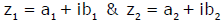are equal if and only if their real & imaginary parts coincide.

C.Conjugate Complex

If z = a + ib then its conjugate complex is obtained by changing the sign of its imaginary part & is denoted by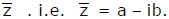Remark :
(i)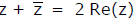(ii)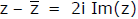(iii)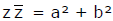which is real
(iv)If z lies in the 1st quadrant then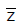lies in the 4th quadrant and -lies in the 2nd quadrant.

Ex.1 Express (1 + 2i)2/(2 + i)2 in the form x + iy.

Sol.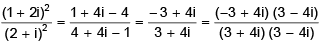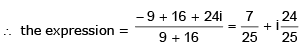Ex.2 Show that a real value of x will satisfy the equation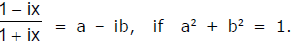Sol.

We have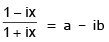or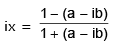[by componendo and dividendo],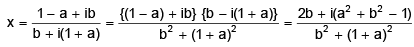Therefore, x will be real, if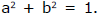Ex.3 Find the square root of a + ib

Sol.

Let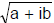= x + iy, where x and y are real. Squaring, a + ib =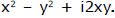Equating real and imaginary parts,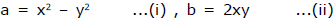Now (x2 + y2)2 = (x2 – y2)2 + 4x2y2 = a2 + b2  or  x+ y2 = √(a2 + b2 )...(iii)

[x and y are real, the sum of their squares must be positive]
From (i) and (iii),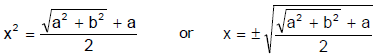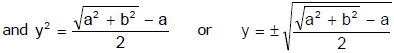If b is positive, both x and y have the same signs and in opposite case, contrary signs. [by (ii)].

The document Algebra of Complex Numbers Notes | Study Mathematics (Maths) Class 11 - JEE is a part of the JEE Course Mathematics (Maths) Class 11.
All you need of JEE at this link: JEEUse Code STAYHOME200 and get INR 200 additional OFF

## Mathematics (Maths) Class 11

154 videos|171 docs|132 tests

Track your progress, build streaks, highlight & save important lessons and more!

,

,

,

,

,

,

,

,

,

,

,

,

,

,

,

,

,

,

,

,

,

;